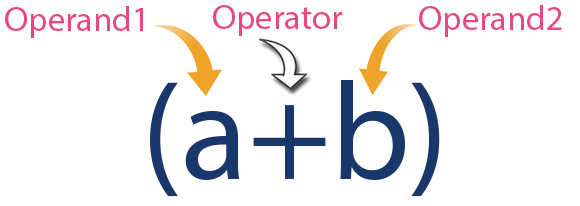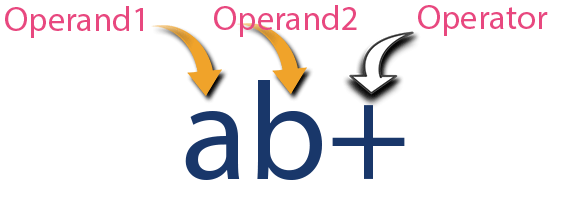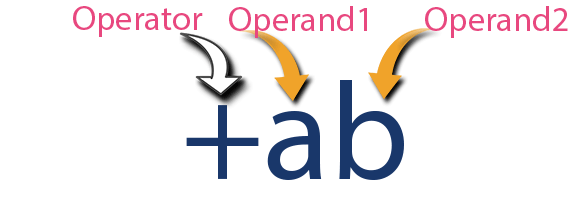The perfect place for easy learning...

×

# Java Expressions

In any programming language, if we want to perform any calculation or to frame any condition etc., we use a set of symbols to perform the task. These set of symbols makes an expression.
In the java programming language, an expression is defined as follows.

An expression is a collection of operators and operands that represents a specific value.

In the above definition, an operator is a symbol that performs tasks like arithmetic operations, logical operations, and conditional operations, etc.
Operands are the values on which the operators perform the task. Here operand can be a direct value or variable or address of memory location.

## Expression Types

In the java programming language, expressions are divided into THREE types. They are as follows.

• Infix Expression
• Postfix Expression
• Prefix Expression

The above classification is based on the operator position in the expression.

### Infix Expression

The expression in which the operator is used between operands is called infix expression.
The infix expression has the following general structure.

Example### Postfix Expression

The expression in which the operator is used after operands is called postfix expression.
The postfix expression has the following general structure.

Example### Prefix Expression

The expression in which the operator is used before operands is called a prefix expression.
The prefix expression has the following general structure.

Example